Updated 26/09/2023
In force

Version from: 28/06/2023
Amendments (1)
There is currently no level 2 legal act based on or specifying Article 259.
Search within this legal act

### Article 259 - Calculation of risk-weighted exposure amounts under the SEC-IRBA

Article 259

Calculation of risk-weighted exposure amounts under the SEC-IRBA

1.

Under the SEC-IRBA, the risk-weighted exposure amount for a securitisation position shall be calculated by multiplying the exposure value of the position calculated in accordance with Article 248 by the applicable risk weight determined as follows, in all cases subject to a floor of 15 %:

 RW = 1 250  % when D ≤ KIRB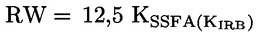when A ≥ KIRB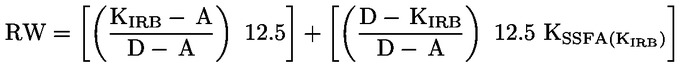when A < KIRB < D

where:

 KIRB is the capital charge of the pool of underlying exposures as defined in Article 255
 D is the detachment point as determined in accordance with Article 256
 A is the attachment point as determined in accordance with Article 256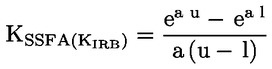where:

 a = – (1/(p * KIRB))
 u = D – KIRB
 l = max (A – KIRB; 0)

where: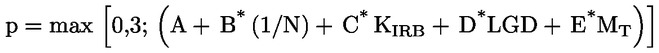where:

 N is the effective number of exposures in the pool of underlying exposures, calculated in accordance with paragraph 4;
 LGD is the exposure-weighted average loss-given-default of the pool of underlying exposures, calculated in accordance with paragraph 5;
 MT is the maturity of the tranche as determined in accordance with Article 257.

The parameters A, B, C, D, and E shall be determined according to the following look-up table:

 A B C D E Non-retail Senior, granular (N ≥ 25) 0 3,56 -1,85 0,55 0,07 Senior, non-granular (N < 25) 0,11 2,61 -2,91 0,68 0,07 Non-senior, granular (N ≥ 25) 0,16 2,87 -1,03 0,21 0,07 Non-senior, non-granular (N < 25) 0,22 2,35 -2,46 0,48 0,07 Retail Senior 0 0 -7,48 0,71 0,24 Non-senior 0 0 -5,78 0,55 0,27
2.
If the underlying IRB pool comprises both retail and non-retail exposures, the pool shall be divided into one retail and one non-retail subpool and, for each subpool, a separate p-parameter (and the corresponding input parameters N, KIRB and LGD) shall be estimated. Subsequently, a weighted average p-parameter for the transaction shall be calculated on the basis of the p-parameters of each subpool and the nominal size of the exposures in each subpool.
3.
Where an institution applies the SEC-IRBA to a mixed pool, the calculation of the p-parameter shall be based on the underlying exposures subject to the IRB Approach only. The underlying exposures subject to the Standardised Approach shall be ignored for these purposes.
4.

The effective number of exposures (N) shall be calculated as follows: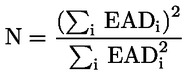where EADi represents the exposure value associated with the ith exposure in the pool.

Multiple exposures to the same obligor shall be consolidated and treated as a single exposure.

5.

The exposure-weighted average LGD shall be calculated as follows: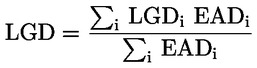where LGDi represents the average LGD associated with all exposures to the ith obligor.

Where credit and dilution risks for purchased receivables are managed in an aggregate manner in a securitisation, the LGD input shall be construed as a weighted average of the LGD for credit risk and 100 % LGD for dilution risk. The weights shall be the stand-alone IRB Approach capital requirements for credit risk and dilution risk, respectively. For these purposes, the presence of a single reserve fund or overcollateralisation available to cover losses from either credit or dilution risk may be regarded as an indication that these risks are managed in an aggregate manner.

6.

Where the share of the largest underlying exposure in the pool (C1) is no more than 3 %, institutions may use the following simplified method to calculate N and the exposure-weighted average LGDs: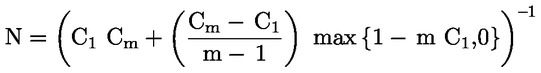LGD = 0,50

where

 Cm denotes the share of the pool corresponding to the sum of the largest m exposures; and
 m is set by the institution.

If only C1 is available and this amount is no more than 0,03, then the institution may set LGD as 0,50 and N as 1/C1.

7.

Where the position is backed by a mixed pool and the institution is able to calculate KIRB on at least 95 % of the underlying exposure amounts in accordance with point (a) of Article 258(1), the institution shall calculate the capital charge for the pool of underlying exposures as: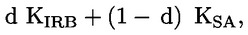where

d is the share of the exposure amount of underlying exposures for which the institution can calculate KIRB over the exposure amount of all underlying exposures.

8.
Where an institution has a securitisation position in the form of a derivative to hedge market risks, including interest rate or currency risks, the institution may attribute to that derivative an inferred risk weight equivalent to the risk weight of the reference position calculated in accordance with this Article.

For the purposes of the first subparagraph, the reference position shall be the position that is pari passu in all respects to the derivative or, in the absence of such pari passu position, the position that is immediately subordinate to the derivative.